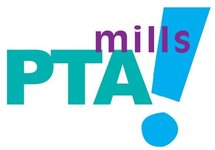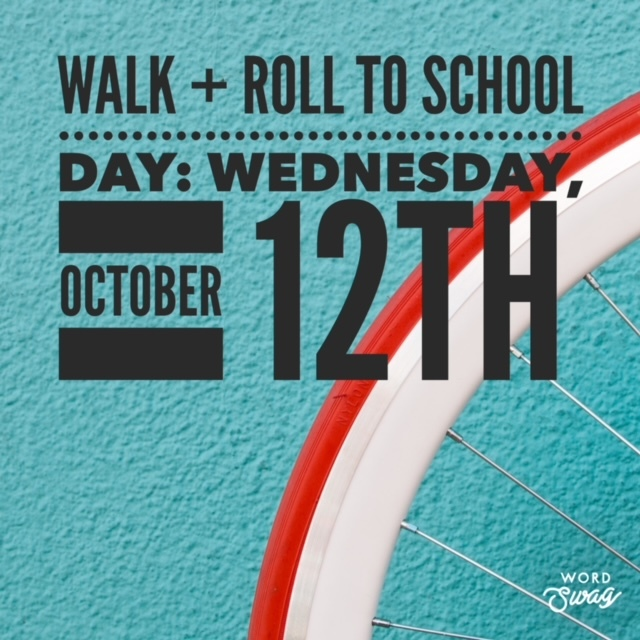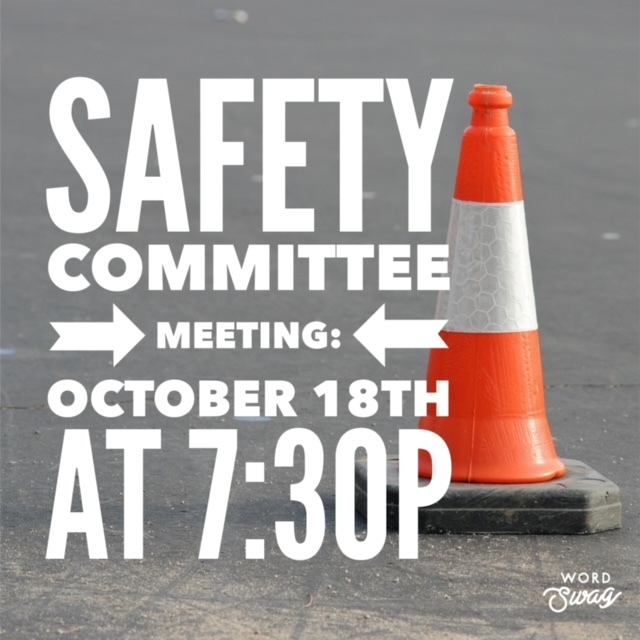## ORDER YOUR MILLS SPIRITWEAR! NOW THROUGH TUESDAY, OCTOBER 11

 table.module-2{width:67.55%;padding:0}table div table+table+table div table{width:67.55%;float:none;margin-left:auto;margin-right:auto;padding:0}table div table+table+table div table a{border:0 none;text-decoration:none}table div table+table+table div table img{width:100%!important;border:0 none;text-decoration:none}table div table+table+table div table td{width:100%;padding:0}/* styles */
 table div table+table+table+table div table{width:100%;padding:0}table div table+table+table+table div table img{width:96.23%;padding:0;float:none}table div table+table+table+table div table td{width:100%;padding:0 1.88% 18px}/* styles */## Walk + Roll to School Day: October 12thMake plans now to walk, bike, or roll to school on Wednesday, October 12th! Create healthy habits, reduce traffic around Mills, and promote a cleaner environment. Join us!

 table div table+table+table+table+table+table+table div table{width:100%;padding:0}table div table+table+table+table+table+table+table div table img{width:96.23%;padding:0;float:none}table div table+table+table+table+table+table+table div table td{width:100%;padding:0 1.88% 18px}/* styles */## October Spirit Nights

 table div table+table+table+table+table+table+table+table+table div table,table.module-8{width:43.4%;float:left;padding:0}table div table+table+table+table+table+table+table+table+table div table a{border:0 none;text-decoration:none}table div table+table+table+table+table+table+table+table+table div table img{width:100%!important;border:0 none;text-decoration:none}table div table+table+table+table+table+table+table+table+table div table td{width:100%;padding:0 20px 20px 0}/* styles */ SAVE THE DATE! We have 2 amazing Spirit Nights planned for October! Wednesday, October 12 - DoubleDave's Pizzaworks - Arbor Trails It covers all sales between 5:00 -8 PM. Dine in, carry out or Delivery. No need to mention Mills. Wednesday, October 19 - Chipotle Mexican Grill - 5000 W Slaughter Ln 4:00-8:00pm Dine in, Drive thru or order online. online ordering code is: YEZF83G. Make sure you show the fundraiser flyer on your phone or tell the cashier at checkout that you are there for a fundraiser for Mills before you pay! If you don’t tell the cashier, Mills won’t get credit for the sale.
 table div table+table+table+table+table+table+table+table+table+table div table{width:100%;padding:0}table div table+table+table+table+table+table+table+table+table+table div table img{width:96.23%;padding:0;float:none}table div table+table+table+table+table+table+table+table+table+table div table td{width:100%;padding:0 1.88% 18px}/* styles */## BEWARE 5th Grade Monster Mash is here!

 table div table+table+table+table+table+table+table+table+table+table+table+table+table+table div table{width:100%;padding:0}table div table+table+table+table+table+table+table+table+table+table+table+table+table+table div table img{width:96.23%;padding:0;float:none}table div table+table+table+table+table+table+table+table+table+table+table+table+table+table div table td{width:100%;padding:0 1.88% 18px}/* styles */## PTA Membership Raffle Winners!

 table div table+table+table+table+table+table+table+table+table+table+table+table+table+table+table+table div table,table.module-15{width:46.98%;float:right;padding:0}table div table+table+table+table+table+table+table+table+table+table+table+table+table+table+table+table div table a{border:0 none;text-decoration:none}table div table+table+table+table+table+table+table+table+table+table+table+table+table+table+table+table div table img{width:100%!important;border:0 none;text-decoration:none}table div table+table+table+table+table+table+table+table+table+table+table+table+table+table+table+table div table td{width:100%;padding:0 0 20px 20px}/* styles */ Wow, Mills!!! We had an incredible week for membership - thank you for your support! We now have 362 members of the Mills PTA and have earned both a schoolwide PJ day and a front office staff funny hair/hat day! The winners of our raffle have been contacted via email. It's not too late if you haven't joined the PTA, we welcome new members throughout the school year. Join here: https://www.millspta.org/product/mills-pta-membership/
 table div table+table+table+table+table+table+table+table+table+table+table+table+table+table+table+table+table div table{width:100%;padding:0}table div table+table+table+table+table+table+table+table+table+table+table+table+table+table+table+table+table div table img{width:96.23%;padding:0;float:none}table div table+table+table+table+table+table+table+table+table+table+table+table+table+table+table+table+table div table td{width:100%;padding:0 1.88% 18px}/* styles */## Family Math Night: Volunteers Needed

 table div table+table+table+table+table+table+table+table+table+table+table+table+table+table+table+table+table+table+table div table,table.module-18{width:49.62%;float:left;padding:0}table div table+table+table+table+table+table+table+table+table+table+table+table+table+table+table+table+table+table+table div table a{border:0 none;text-decoration:none}table div table+table+table+table+table+table+table+table+table+table+table+table+table+table+table+table+table+table+table div table img{width:100%!important;border:0 none;text-decoration:none}table div table+table+table+table+table+table+table+table+table+table+table+table+table+table+table+table+table+table+table div table td{width:100%;padding:0 20px 20px 0}/* styles */ The PTA will be hosting a Family Math Night on Wednesday, November 9th, and we need volunteers! As part of Math Night, families can travel through a variety of math games provided by the event leader, Mathnasium, and volunteers will help us teach and play the games. We love parent volunteers, but this is also a great volunteer opportunity for older siblings who need service hours. Please sign up here: https://www.signupgenius.com/go/10c0d4bacae23a4f58-mills
 table div table+table+table+table+table+table+table+table+table+table+table+table+table+table+table+table+table+table+table+table div table{width:100%;padding:0}table div table+table+table+table+table+table+table+table+table+table+table+table+table+table+table+table+table+table+table+table div table img{width:96.23%;padding:0;float:none}table div table+table+table+table+table+table+table+table+table+table+table+table+table+table+table+table+table+table+table+table div table td{width:100%;padding:0 1.88% 18px}/* styles */## PTA Safety Committee MeetingJoin us for our first PTA Safety committee of the year on Tuesday, October 18th at 7:30pm via Zoom. (Zoom link is below!) At this meeting, we will brainstorm ways to address safety concerns at Mills. The primary discussion will be about traffic and pedestrian safety, but we can discuss other safety concerns as well. If you have questions, please email me at christy.millspta@gmail.com. Hope to see you there!

Zoom info:
Topic: PTA Safety Committee
Time: Oct 18, 2022 07:30 PM Central Time (US and Canada)

Meeting ID: 822 9311 4330
Passcode: 188202

 table div table+table+table+table+table+table+table+table+table+table+table+table+table+table+table+table+table+table+table+table+table+table+table div table{width:100%;padding:0}table div table+table+table+table+table+table+table+table+table+table+table+table+table+table+table+table+table+table+table+table+table+table+table div table img{width:96.23%;padding:0;float:none}table div table+table+table+table+table+table+table+table+table+table+table+table+table+table+table+table+table+table+table+table+table+table+table div table td{width:100%;padding:0 1.88% 18px}/* styles */## Connect with the PTA

 /* styles */ Please join our PTA Facebook group! Lots of PTA info is shared on Facebook, and we don't want you to miss it! (Much of the same info is shared here in our Mills Messenger, but it comes to Facebook more quickly and often offers realtime reminders!) The group name is Joe Dan Mills Elementary Families & Staff Austin, TX, and you'll be asked a few questions to join.
 table div table+table+table+table+table+table+table+table+table+table+table+table+table+table+table+table+table+table+table+table+table+table+table+table+table+table div table{width:100%;padding:0}table div table+table+table+table+table+table+table+table+table+table+table+table+table+table+table+table+table+table+table+table+table+table+table+table+table+table div table img{width:96.23%;padding:0;float:none}table div table+table+table+table+table+table+table+table+table+table+table+table+table+table+table+table+table+table+table+table+table+table+table+table+table+table div table td{width:100%;padding:0 1.88% 18px}/* styles */## Affiliate NewsFall Youth Academy: 6-week youth volleyball camp for girls and boys!
 table div table+table+table+table+table+table+table+table+table+table+table+table+table+table+table+table+table+table+table+table+table+table+table+table+table+table+table+table+table div table{width:100%;padding:0}table div table+table+table+table+table+table+table+table+table+table+table+table+table+table+table+table+table+table+table+table+table+table+table+table+table+table+table+table+table div table img{width:96.23%;padding:0;float:none}table div table+table+table+table+table+table+table+table+table+table+table+table+table+table+table+table+table+table+table+table+table+table+table+table+table+table+table+table+table div table td{width:100%;padding:0 1.88% 18px}/* styles */## Need to get your message out?

 /* styles */ Submit info to the Mills Messenger. Want to see the Mills Calendar on your Google calendar? Add Mills Calendar to my Google Calendar. Have a friend who isn't getting the Messenger but wants to? Send them this link to sign up: https://madmimi.com/signups/join/7059
 table div table+table+table+table+table+table+table+table+table+table+table+table+table+table+table+table+table+table+table+table+table+table+table+table+table+table+table+table+table+table+table+table div table{width:100%;padding:0}table div table+table+table+table+table+table+table+table+table+table+table+table+table+table+table+table+table+table+table+table+table+table+table+table+table+table+table+table+table+table+table+table div table img{width:96.23%;padding:0;float:none}table div table+table+table+table+table+table+table+table+table+table+table+table+table+table+table+table+table+table+table+table+table+table+table+table+table+table+table+table+table+table+table+table div table td{width:100%;padding:0 1.88% 18px}/* styles *//* styles */ Find recent editions of the Mills Messenger at millspta.org.